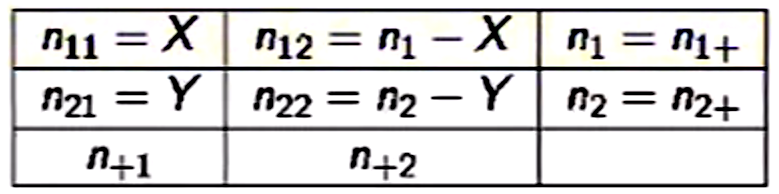### $$\chi^2$$ TEST of PROPORTIONS IS IDENTICAL TO $$Z$$-TEST SQUARED:

This entry corresponds to this question on CV.

BACKGROUND:

The opening of this paper reads:

Karl Pearson’s famous chi-square contingency test is derived from another statistic, called the z statistic, based on the Normal distribution. The simplest versions of $$\chi^2$$ can be shown to be mathematically identical to equivalent z tests. The tests produce the same result in all circumstances. For all intents and purposes “chi-squared” could be called “z-squared”. The critical values of $$\chi^2$$ for one degree of freedom are the square of the corresponding critical values of z.

This has been asserted multiple times in CV (here, here, here and others).

And indeed we can prove that $$\chi^2_{1\,df}$$ is equivalent to $$X^2$$ with $$X\sim N(0,1)$$:

Let’s say that $$X \sim N(0,1)$$ and that $$Y=X^2$$ and find the density of $$Y$$ by using the $$cdf$$ method:

$$p(Y \leq y) = p(X^2 \leq y)= p(-\sqrt{y} \leq x \leq \sqrt{y})$$. The problem is that we cannot integrate in close form the density of the normal distribution. But we can express it:

$F_X(y) = F_X(\sqrt{y})- F_X(-\sqrt[]{y}).$ Taking the derivative:

$f_X(y)= F_X'(\sqrt{y})\,\frac{1}{2\sqrt{y}}+ F_X'(\sqrt{-y})\,\frac{1}{2\sqrt{y}}.$

Since the values of the normal $$pdf$$ are symmetrical:

$$f_X(y)= F_X'(\sqrt{y})\,\frac{1}{\sqrt{y}}$$. Equating this to the $$pdf$$ of the normal (now the $$x$$ in the $$pdf$$ will be $$\sqrt{y}$$ to be plugged into the $$e^{-\frac{x^2}{2}}$$ part of the normal $$pdf$$); and remembering to in include $$\frac{1}{\sqrt{y}}$$ at the end:

$f_X(y)= F_X'(\sqrt[]{y})\,\frac{1}{\sqrt[]{y}}= \frac{1}{\sqrt{2\pi}}\,e^{-\frac{y}{2}}\, \frac{1}{\sqrt[]{y}}=\frac{1}{\sqrt{2\pi}}\,e^{-\frac{y}{2}}\, y^{\frac{1}{2}- 1}$

Compare to the pdf of the chi square:

$f_X(x)= \frac{1}{2^{\nu/2}\Gamma(\frac{\nu}{2})}e^{\frac{-x}{2}}x^{\frac{\nu}{2}-1}$

Since $$\Gamma(1/2)=\sqrt{\pi}$$, for $$1$$ df, we have derived exactly the $$pdf$$ of the chi square.

Further, if we call the function prop.test() in R we are invoking the same $$\chi^2$$ test as if we decide upon chisq.test().

THE QUESTION:

So I get all these points, yet I still don’t know how they apply to the actual implementation of these two tests for two reasons:

1. A z-test is not squared.

2. The actual test statistics are completely different:

The value of the test-statistic for a $$\chi^2$$ is:

$$\large \chi^2 = \displaystyle \sum_{i=1}^{n} \frac{(O_i - E_i)^2}{E_i} = \,N\, \displaystyle \sum_{i=1}^n \,p_i\, \left(\frac{\frac{O_i}{N} - p_i}{p_i}\right)^2= \frac{n(n_{11}\,n_{22}\,-\,n_{12}\,n_{21})^2}{n_{+1}\,n_{+2}\,n_{1+}\,n_{2+}}$$
where

$$\chi^2$$ = Pearson’s cumulative test statistic, which asymptotically approaches a $$\chi^2$$ distribution. $$O_i$$ = the number of observations of type $$i$$; $$N$$ = total number of observations; $$E_i$$ = $$N p_i$$ = the expected (theoretical) frequency of type $$i$$, asserted by the null hypothesis that the fraction of type $$i$$ in the population is $$p_i$$; $$n$$ = the number of cells in the table.

Of note the last expression of the $$\chi^2$$ statistic applies to $$2$$x$$2$$ tables and the nomenclature is as follows:On the other hand, the test statistic for a $$z$$-test is:

$$\large \displaystyle Z = \frac{\frac{x_1}{n_1}-\frac{x_2}{n_2}}{\sqrt{p\,(1-p)(1/n_1+1/n_2)}}$$ with $$\displaystyle p = \frac{x_1\,+\,x_2}{n_1\,+\,n_2}$$, where $$x_1$$ and $$x_2$$ are the number of “successes”, over the number of subjects in each one of the levels of the categorical variables, i.e. $$n_1$$ and $$n_2$$.

This formula seems to rely on the binomial distribution.

These two tests statistics are clearly different, and result in different results for the actual test statistics, as well as for the p-values: 5.8481 for the $$\chi^2$$ and 2.4183 for the z-test. The p-value for the $$\chi^2$$ test is 0.01559, while for the z-test is 0.0077. The difference explained by two-tailed versus one-tailed: $$\small 0.01559/2=0.007795$$.

In the example on the last hyperlink the $$\chi^2$$ is almost the square of the z-test statistic, but not quite, and the p-values are different.

Performing the chisq.test() with correct=FALSE yields exactly the square of the z-test: in the output numbers included in the end of the question $$\small 2.4183^2=5.84817$$.

These are two identical tests. $$Z$$ squared is the chi-square statistic. Let you have $$2x2$$ frequency table where columns are the two groups and the rows are “success” and “failure”. Then the so called expected frequencies of the chi-square test in a given column is the weighted (by the groups’ $$N$$) average column (group) profile multiplied by that group’s $$N$$. Thus, it comes that chi-square tests the deviation of each of the two groups profiles from this average group profile - which is equivalent to testing the groups’ profiles difference from each other, the $$z$$-test of proportions.

Let us have a $$2x2$$ frequency table where columns are two groups of respondents and rows are the two responses “Yes” and “No”. And we’ve turned the frequencies into the proportions within group, i.e. into the vertical profiles:

      Gr1   Gr2  Total
Yes   p1    p2     p
No    q1    q2     q
--------------
100%  100%   100%
n1    n2     N

The usual (not Yates corrected) $$\chi^2$$ of this table, after you substitute proportions instead of frequencies in its formula, looks like this:

$n_1\left[\frac{(p_1-p)^2}{p}+\frac{(q_1-q)^2}{q}\right]+n_2\left[\frac{(p_2-p)^2}{p}+\frac{(q_2-q)^2}{q}\right]= \frac{n_1(p_1-p)^2+n_2(p_2-p)^2}{pq}.$

Remember that $$p= \frac{n_1p_1+n_2p_2}{n_1+n_2}$$, the element of the weighted average profile of the two profiles (p1,q1) and (p2,q2), and plug it in the formula, to obtain

$...= \frac{(p_1-p_2)^2(n_1^2n_2+n_1n_2^2)}{pqN^2}$

Divide both numerator and denominator by the $$(n_1^2n_2+n_1n_2^2)$$ and get $\frac{(p_1-p_2)^2}{pq(1/n_1+1/n_2)}=Z^2,$

the squared z-statistic of the z-test of proportions for “Yes” response.

Thus, the $$2x2$$ homogeneity Chi-square statistic (and test) is equivalent to the z-test of two proportions. The so called expected frequencies computed in the chi-square test in a given column is the weighted (by the group n) average vertical profile (i.e. the profile of the “average group”) multiplied by that group’s n. Thus, it comes out that chi-square tests the deviation of each of the two groups profiles from this average group profile, - which is equivalent to testing the groups’ profiles difference from each other, which is the z-test of proportions.

This is one demonstration of a link between a variables association measure (chi-square) and a group difference measure (z-test statistic). Attribute associations and group differences are (often) the two facets of the same thing.

Showing the expansion in the first line above:

$$\large n_1\left[\frac{(p_1-p)^2}{p}+\frac{(q_1-q)^2}{q}\right]+n_2\left[\frac{(p_2-p)^2}{p}+\frac{(q_2-q)^2}{q}\right] = \frac{n_1(p_1-p)^2q}{pq}+\frac{n_1(q_1-q)^2p}{pq}+\frac{n_2(p_2-p)^2q}{pq}+\frac{n_2(q_2-q)^2p}{pq}$$

$$\large= \frac{n_1(p_1-p)^2(1-p)+n_1(1-p_1-1+p)^2p+n_2(p_2-p)^2(1-p)+n_2(1-p_2-1+p)^2p}{pq}$$

$$\large= \frac{n_1(p_1-p)^2(1-p)+n_1(p-p_1)^2p+n_2(p_2-p)^2(1-p)+n_2(p-p_2)^2p}{pq} = \frac{[n_1(p_1-p)^2][(1-p)+p]+[n_2(p_2-p)^2][(1-p)+p]}{pq} = \frac{n_1(p_1-p)^2+n_2(p_2-p)^2}{pq}.$$